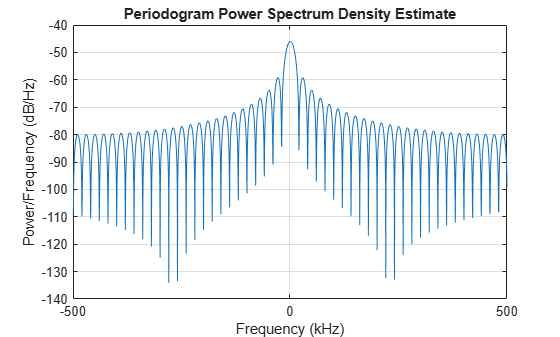# getStretchProcessor

System object: phased.LinearFMWaveform
Package: phased

Create stretch processor for waveform

## Syntax

```HS = getStretchProcessor(H) HS = getStretchProcessor(H,refrng) HS = getStretchProcessor(H,refrng,rngspan) HS = getStretchProcessor(H,refrng,rngspan,v) ```

## Description

`HS = getStretchProcessor(H)` returns the stretch processor for the waveform, `H`. `HS` is set up so the reference range corresponds to 1/4 of the maximum unambiguous range of a pulse. The range span corresponds to 1/10 of the distance traveled by the wave within the pulse width. The propagation speed is the speed of light.

`HS = getStretchProcessor(H,refrng)` specifies the reference range.

`HS = getStretchProcessor(H,refrng,rngspan)` specifies the range span. The reference interval is centered at `refrng`.

`HS = getStretchProcessor(H,refrng,rngspan,v)` specifies the propagation speed.

## Input Arguments

 `H` Linear FM pulse waveform object. `refrng` Reference range, in meters, as a positive scalar. Default: 1/4 of the maximum unambiguous range of a pulse `rngspan` Length of the interval of ranges of interest, in meters, as a positive scalar. The center of the interval is the range value specified in the `refrng` argument. Default: 1/10 of the distance traveled by the wave within the pulse width `v` Propagation speed, in meters per second, as a positive scalar. Default: Speed of light

## Output Arguments

 `HS` Stretch processor as a `phased.StretchProcessor` System object™.

## Examples

expand all

Use stretch processing to locate a target at a range of 4950 m.

Note: This example runs only in R2016b or later. If you are using an earlier release, replace each call to the function with the equivalent `step` syntax. For example, replace `myObject(x)` with `step(myObject,x)`.

Simulate the signal.

```waveform = phased.LinearFMWaveform; x = waveform(); c = physconst('LightSpeed'); rng = 4950.0; num_samples = round(rng/(c/(2*waveform.SampleRate))); x = circshift(x,num_samples);```

Perform stretch processing.

```stretchproc = getStretchProcessor(waveform,5000,200,c); y = stretchproc(x);```

Plot the spectrum of the resulting signal.

```[Pxx,F] = periodogram(y,[],2048,stretchproc.SampleRate,'centered'); plot(F/1000,10*log10(Pxx)) grid xlabel('Frequency (kHz)') ylabel('Power/Frequency (dB/Hz)') title('Periodogram Power Spectrum Density Estimate')```Detect the range.

```[~,rngidx] = findpeaks(pow2db(Pxx/max(Pxx)),'MinPeakHeight',-5); rngfreq = F(rngidx); rng = stretchfreq2rng(rngfreq,stretchproc.SweepSlope,stretchproc.ReferenceRange,c)```
```rng = 4.9634e+03 ```